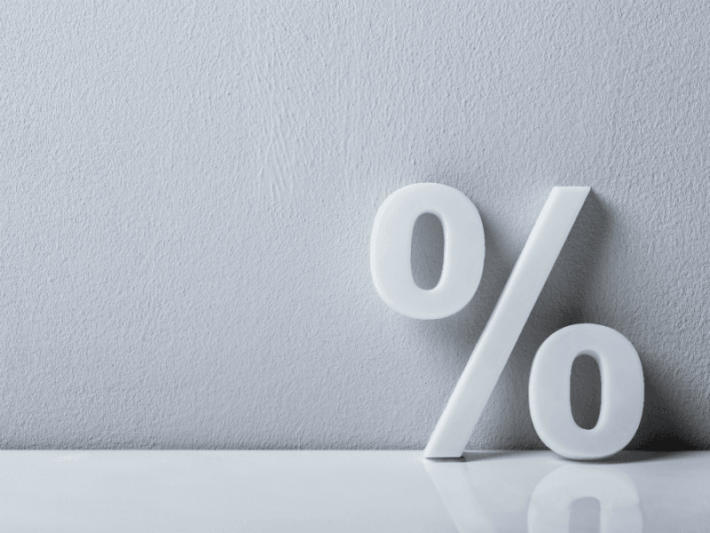ôô

# How to Quickly Calculate PercentagesHow to calculate percentages is easier than you think. Quick, what’s 36% of 25? Or how about 250% of 20? Learn a quick and dirty tip to help you calculate all of those pesky percentages in your head.

By
Jason Marshall, PhD
Episode #127## How to Calculate Percentages > 100%

What about the second example I gave earlier: 250% of 20? According to our rule, 250% of 20 must be the same as 20% of 250. Since 20% is just 2 x 10%, and since we know that it’s easy to find 10% of any number by dividing it by 10, we see that 10% of 250 is 250/10 = 25, and therefore that 20% of 250 must be equal to 2 x 25 = 50. According to the handy dandy rule we’ve just proven to ourselves, the fact that 20% of 250 is equal to 50 means that 250% of 20 must be equal to 50 as well.

But wait a minute. What exactly does it mean that 250% of 20 is equal to 50? And, more broadly, what do percentages greater than 100 signify in the first place? Well, just as something like 36% is equivalent to the fraction 36/100, it’s also true that something like 250% is equivalent to the fraction 250/100. In other words, the meaning of “per-cent” hasn’t changed, so 250% still means 250 per 100. So, just as we found earlier, 250% of 20 is equal to 250/100 x 20 = 2.5 x 20 which is 50.

## What Do Percentages > 100% Mean?

There’s a common misconception that it doesn’t make sense to talk about percentages that are greater than 100. After all, you can’t have more than a whole of anything, right? Wrong—of course you can! It’s perfectly reasonable to talk about 1.5 somethings (which is the same as 150% of that something), or 2 somethings (which is the same as 200% of that something), or any other number of somethings.

Just remember: Anything less than 100% of a number will always be a number that’s smaller than the original; anything greater than 100% of a number will always be a number that’s larger than the original.

## Wrap Up

Okay, that’s all the math we have time for today. In the meantime, remember to become a fan of the Math Dude on Facebook where you’ll find lots of great math posted throughout the week. If you’re on Twitter, please follow me there, too. Finally, please send your math questions my way via Facebook, Twitter, or email at mathdude@quickanddirtytips.com.

Until next time, this is Jason Marshall with The Math Dude’s Quick and Dirty Tips to Make Math Easier. Thanks for reading, math fans!

Percent image from Shutterstock

## Pages